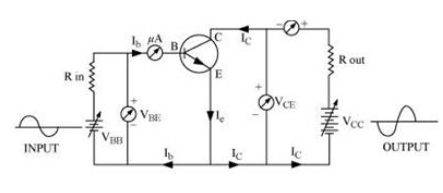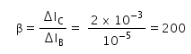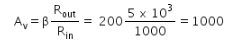# Draw a circuit diagram for use of NPN transistor as an amplifier in common

Draw a circuit diagram for use of NPN transistor as an amplifier in common emitter configuration. The input resistance of a transistor is 1000 Ω. On changing its base current by 10 µA. the collector current increases by 2 mA.
If a load resistance of 5 k Ω is used in the circuit, calculate:
(i) The current gain
(ii) Voltage gain of the amplifierNumerical: Given
Rin = 1000 Ω
ΔIB = 10 μA = 10-5 A
ΔIC = 2mA = 2 x 10-3A
Rout = 5 kΩ = 5 x 103 Ω
(i) Current gain(ii) Voltage gain